# Iterative Tower of Hanoi

Tower of Hanoi is a mathematical puzzle. It consists of three poles and a number of disks of different sizes which can slide onto any poles. The puzzle starts with the disk in a neat stack in ascending order of size in one pole, the smallest at the top thus making a conical shape. The objective of the puzzle is to move all the disks from one pole (say ‘source pole’) to another pole (say ‘destination pole’) with the help of the third pole (say auxiliary pole).

The puzzle has the following two rules:
1. You can’t place a larger disk onto smaller disk
2. Only one disk can be moved at a time

We’ve already discussed recursive solution for Tower of Hanoi. We have also seen that, for n disks, total 2n – 1 moves are required.

Iterative Algorithm:

```1. Calculate the total number of moves required i.e. "pow(2, n)
- 1" here n is number of disks.
2. If number of disks (i.e. n) is even then interchange destination
pole and auxiliary pole.
3. for i = 1 to total number of moves:
if i%3 == 1:
legal movement of top disk between source pole and
destination pole
if i%3 == 2:
legal movement top disk between source pole and
auxiliary pole
if i%3 == 0:
legal movement top disk between auxiliary pole
and destination pole
```

Example:

```Let us understand with a simple example with 3 disks:
So, total number of moves required = 7
```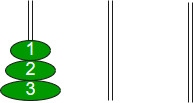```        S                      A                   D

When i= 1, (i % 3 == 1) legal movement between‘S’ and ‘D’
```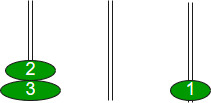```When i = 2,  (i % 3 == 2) legal movement between ‘S’ and ‘A’

```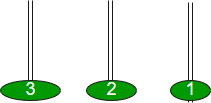```When i = 3, (i % 3 == 0) legal movement between ‘A’ and ‘D’ ’
```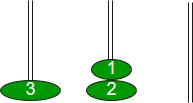```When i = 4, (i % 3 == 1) legal movement between ‘S’ and ‘D’
```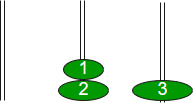```When i = 5, (i % 3 == 2) legal movement between ‘S’ and ‘A’
```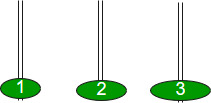```When i = 6, (i % 3 == 0) legal movement between ‘A’ and ‘D’
```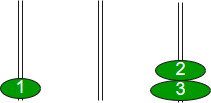```When i = 7, (i % 3 == 1) legal movement between ‘S’ and ‘D’
```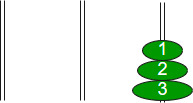So, after all these destination poles contains all the in order of size.
After observing above iterations, we can think that after a disk other than the smallest disk is moved, the next disk to be moved must be the smallest disk because it is the top disk resting on the spare pole and there are no other choices to move a disk.

## C++

 `// C Program for Iterative Tower of Hanoi ` `#include ` `#include ` `#include ` `#include `   `// A structure to represent a stack ` `struct` `Stack ` `{ ` `unsigned capacity; ` `int` `top; ` `int` `*array; ` `}; `   `// function to create a stack of given capacity. ` `struct` `Stack* createStack(unsigned capacity) ` `{ ` `    ``struct` `Stack* stack = ` `        ``(``struct` `Stack*) ``malloc``(``sizeof``(``struct` `Stack)); ` `    ``stack -> capacity = capacity; ` `    ``stack -> top = -1; ` `    ``stack -> array = ` `        ``(``int``*) ``malloc``(stack -> capacity * ``sizeof``(``int``)); ` `    ``return` `stack; ` `} `   `// Stack is full when top is equal to the last index ` `int` `isFull(``struct` `Stack* stack) ` `{ ` `return` `(stack->top == stack->capacity - 1); ` `} `   `// Stack is empty when top is equal to -1 ` `int` `isEmpty(``struct` `Stack* stack) ` `{ ` `return` `(stack->top == -1); ` `} `   `// Function to add an item to stack. It increases ` `// top by 1 ` `void` `push(``struct` `Stack *stack, ``int` `item) ` `{ ` `    ``if` `(isFull(stack)) ` `        ``return``; ` `    ``stack -> array[++stack -> top] = item; ` `} `   `// Function to remove an item from stack. It ` `// decreases top by 1 ` `int` `pop(``struct` `Stack* stack) ` `{ ` `    ``if` `(isEmpty(stack)) ` `        ``return` `INT_MIN; ` `    ``return` `stack -> array[stack -> top--]; ` `} `   `//Function to show the movement of disks ` `void` `moveDisk(``char` `fromPeg, ``char` `toPeg, ``int` `disk) ` `{ ` `    ``printf``(``"Move the disk %d from \'%c\' to \'%c\'\n"``, ` `        ``disk, fromPeg, toPeg); ` `}`   `// Function to implement legal movement between ` `// two poles ` `void` `moveDisksBetweenTwoPoles(``struct` `Stack *src, ` `            ``struct` `Stack *dest, ``char` `s, ``char` `d) ` `{ ` `    ``int` `pole1TopDisk = pop(src); ` `    ``int` `pole2TopDisk = pop(dest); `   `    ``// When pole 1 is empty ` `    ``if` `(pole1TopDisk == INT_MIN) ` `    ``{ ` `        ``push(src, pole2TopDisk); ` `        ``moveDisk(d, s, pole2TopDisk); ` `    ``} `   `    ``// When pole2 pole is empty ` `    ``else` `if` `(pole2TopDisk == INT_MIN) ` `    ``{ ` `        ``push(dest, pole1TopDisk); ` `        ``moveDisk(s, d, pole1TopDisk); ` `    ``} `   `    ``// When top disk of pole1 > top disk of pole2 ` `    ``else` `if` `(pole1TopDisk > pole2TopDisk) ` `    ``{ ` `        ``push(src, pole1TopDisk); ` `        ``push(src, pole2TopDisk); ` `        ``moveDisk(d, s, pole2TopDisk); ` `    ``} `   `    ``// When top disk of pole1 < top disk of pole2 ` `    ``else` `    ``{ ` `        ``push(dest, pole2TopDisk); ` `        ``push(dest, pole1TopDisk); ` `        ``moveDisk(s, d, pole1TopDisk); ` `    ``} ` `} `   `//Function to implement TOH puzzle ` `void` `tohIterative(``int` `num_of_disks, ``struct` `Stack ` `            ``*src, ``struct` `Stack *aux, ` `            ``struct` `Stack *dest) ` `{ ` `    ``int` `i, total_num_of_moves; ` `    ``char` `s = ``'S'``, d = ``'D'``, a = ``'A'``; `   `    ``//If number of disks is even, then interchange ` `    ``//destination pole and auxiliary pole ` `    ``if` `(num_of_disks % 2 == 0) ` `    ``{ ` `        ``char` `temp = d; ` `        ``d = a; ` `        ``a = temp; ` `    ``} ` `    ``total_num_of_moves = ``pow``(2, num_of_disks) - 1; `   `    ``//Larger disks will be pushed first ` `    ``for` `(i = num_of_disks; i >= 1; i--) ` `        ``push(src, i); `   `    ``for` `(i = 1; i <= total_num_of_moves; i++) ` `    ``{ ` `        ``if` `(i % 3 == 1) ` `        ``moveDisksBetweenTwoPoles(src, dest, s, d); `   `        ``else` `if` `(i % 3 == 2) ` `        ``moveDisksBetweenTwoPoles(src, aux, s, a); `   `        ``else` `if` `(i % 3 == 0) ` `        ``moveDisksBetweenTwoPoles(aux, dest, a, d); ` `    ``} ` `} `   `// Driver Program ` `int` `main() ` `{ ` `    ``// Input: number of disks ` `    ``unsigned num_of_disks = 3; `   `    ``struct` `Stack *src, *dest, *aux; `   `    ``// Create three stacks of size 'num_of_disks' ` `    ``// to hold the disks ` `    ``src = createStack(num_of_disks); ` `    ``aux = createStack(num_of_disks); ` `    ``dest = createStack(num_of_disks); `   `    ``tohIterative(num_of_disks, src, aux, dest); ` `    ``return` `0; ` `} `

## Java

 `// Java program for iterative ` `// Tower of Hanoi` `class` `TOH{` `    `  `// A structure to represent a stack` `class` `Stack` `{` `    ``int` `capacity;` `    ``int` `top;` `    ``int` `array[];` `}`   `// Function to create a stack of given capacity.` `Stack createStack(``int` `capacity)` `{` `    ``Stack stack = ``new` `Stack();` `    ``stack.capacity = capacity;` `    ``stack.top = -``1``;` `    ``stack.array = ``new` `int``[capacity];` `    ``return` `stack;` `}`   `// Stack is full when the top is equal` `// to the last index` `boolean` `isFull(Stack stack)` `{` `    ``return` `(stack.top == stack.capacity - ``1``);` `}`   `// Stack is empty when top is equal to -1` `boolean` `isEmpty(Stack stack)` `{` `    ``return` `(stack.top == -``1``);` `}`   `// Function to add an item to stack.It ` `// increases top by 1` `void` `push(Stack stack, ``int` `item)` `{` `    ``if` `(isFull(stack))` `        ``return``;` `        `  `    ``stack.array[++stack.top] = item;` `}`   `// Function to remove an item from stack.It` `// decreases top by 1` `int` `pop(Stack stack)` `{` `    ``if` `(isEmpty(stack))` `        ``return` `Integer.MIN_VALUE;` `        `  `    ``return` `stack.array[stack.top--];` `}`   `// Function to implement legal movement between` `// two poles` `void` `moveDisksBetweenTwoPoles(Stack src, Stack dest, ` `                              ``char` `s, ``char` `d) ` `{` `    ``int` `pole1TopDisk = pop(src);` `    ``int` `pole2TopDisk = pop(dest);`   `    ``// When pole 1 is empty` `    ``if` `(pole1TopDisk == Integer.MIN_VALUE) ` `    ``{` `        ``push(src, pole2TopDisk);` `        ``moveDisk(d, s, pole2TopDisk);` `    ``}` `    `  `    ``// When pole2 pole is empty` `    ``else` `if` `(pole2TopDisk == Integer.MIN_VALUE) ` `    ``{` `        ``push(dest, pole1TopDisk);` `        ``moveDisk(s, d, pole1TopDisk);` `    ``}` `    `  `    ``// When top disk of pole1 > top disk of pole2` `    ``else` `if` `(pole1TopDisk > pole2TopDisk) ` `    ``{` `        ``push(src, pole1TopDisk);` `        ``push(src, pole2TopDisk);` `        ``moveDisk(d, s, pole2TopDisk);` `    ``}` `    ``// When top disk of pole1 < top disk of pole2` `    ``else` `    ``{` `        ``push(dest, pole2TopDisk);` `        ``push(dest, pole1TopDisk);` `        ``moveDisk(s, d, pole1TopDisk);` `    ``}` `}`   `// Function to show the movement of disks` `void` `moveDisk(``char` `fromPeg, ``char` `toPeg, ``int` `disk)` `{` `    ``System.out.println(``"Move the disk "` `+ disk + ` `                            ``" from "` `+ fromPeg + ` `                              ``" to "` `+ toPeg);` `}`   `// Function to implement TOH puzzle` `void` `tohIterative(``int` `num_of_disks, Stack` `                  ``src, Stack aux, Stack dest)` `{` `    ``int` `i, total_num_of_moves;` `    ``char` `s = ``'S'``, d = ``'D'``, a = ``'A'``;` ` `  `    ``// If number of disks is even, then ` `    ``// interchange destination pole and` `    ``// auxiliary pole` `    ``if` `(num_of_disks % ``2` `== ``0``)` `    ``{` `        ``char` `temp = d;` `        ``d = a;` `        ``a  = temp;` `    ``}` `    ``total_num_of_moves = (``int``)(Math.pow(` `                         ``2``, num_of_disks) - ``1``);` ` `  `    ``// Larger disks will be pushed first` `    ``for``(i = num_of_disks; i >= ``1``; i--)` `        ``push(src, i);` ` `  `    ``for``(i = ``1``; i <= total_num_of_moves; i++)` `    ``{` `        ``if` `(i % ``3` `== ``1``)` `          ``moveDisksBetweenTwoPoles(src, dest, s, d);` ` `  `        ``else` `if` `(i % ``3` `== ``2``)` `          ``moveDisksBetweenTwoPoles(src, aux, s, a);` ` `  `        ``else` `if` `(i % ``3` `== ``0``)` `          ``moveDisksBetweenTwoPoles(aux, dest, a, d);` `    ``}` `}`   `// Driver code` `public` `static` `void` `main(String[] args)` `{` `    `  `    ``// Input: number of disks` `    ``int` `num_of_disks = ``3``;` `    `  `    ``TOH ob = ``new` `TOH();` `    ``Stack src, dest, aux;` `    `  `    ``// Create three stacks of size 'num_of_disks'` `    ``// to hold the disks` `    ``src = ob.createStack(num_of_disks);` `    ``dest = ob.createStack(num_of_disks);` `    ``aux = ob.createStack(num_of_disks);` `    `  `    ``ob.tohIterative(num_of_disks, src, aux, dest);` `}` `}`   `// This code is contibuted by Sumit Ghosh`

## C#

 `// C# program for iterative ` `// Tower of Hanoi ` `using` `System;`   `class` `GFG ` `{ ` `    ``// A structure to represent a stack ` `    ``public` `class` `Stack ` `    ``{ ` `        ``public` `int` `capacity; ` `        ``public` `int` `top; ` `        ``public` `int` `[]array; ` `    ``} ` `    `  `    ``// function to create a stack of given capacity. ` `    ``Stack createStack(``int` `capacity) ` `    ``{ ` `        ``Stack stack = ``new` `Stack(); ` `        ``stack.capacity = capacity; ` `        ``stack.top = -1; ` `        ``stack.array = ``new` `int``[capacity]; ` `        ``return` `stack; ` `    ``} ` `    `  `    ``// Stack is full when top is equal to the last index ` `    ``Boolean isFull(Stack stack) ` `    ``{ ` `        ``return` `(stack.top == stack.capacity - 1); ` `    ``} ` `    `  `    ``// Stack is empty when top is equal to -1 ` `    ``Boolean isEmpty(Stack stack) ` `    ``{ ` `        ``return` `(stack.top == -1); ` `    ``} ` `    `  `    ``// Function to add an item to stack. ` `    ``// It increases top by 1 ` `    ``void` `push(Stack stack,``int` `item) ` `    ``{ ` `        ``if``(isFull(stack)) ` `            ``return``; ` `        ``stack.array[++stack.top] = item; ` `    ``} ` `    `  `    ``// Function to remove an item from stack.  ` `    ``// It decreases top by 1 ` `    ``int` `pop(Stack stack) ` `    ``{ ` `        ``if``(isEmpty(stack)) ` `            ``return` `int``.MinValue; ` `        ``return` `stack.array[stack.top--]; ` `    ``} ` `    `  `    ``// Function to implement legal  ` `    ``// movement between two poles ` `    ``void` `moveDisksBetweenTwoPoles(Stack src, Stack dest, ` `                                            ``char` `s, ``char` `d) ` `    ``{ ` `        ``int` `pole1TopDisk = pop(src); ` `        ``int` `pole2TopDisk = pop(dest); `   `        ``// When pole 1 is empty ` `        ``if` `(pole1TopDisk == ``int``.MinValue) ` `        ``{ ` `            ``push(src, pole2TopDisk); ` `            ``moveDisk(d, s, pole2TopDisk); ` `        ``} ` `        `  `        ``// When pole2 pole is empty ` `        ``else` `if` `(pole2TopDisk == ``int``.MinValue) ` `        ``{ ` `            ``push(dest, pole1TopDisk); ` `            ``moveDisk(s, d, pole1TopDisk); ` `        ``} ` `        `  `        ``// When top disk of pole1 > top disk of pole2 ` `        ``else` `if` `(pole1TopDisk > pole2TopDisk) ` `        ``{ ` `            ``push(src, pole1TopDisk); ` `            ``push(src, pole2TopDisk); ` `            ``moveDisk(d, s, pole2TopDisk); ` `        ``} ` `        `  `        ``// When top disk of pole1 < top disk of pole2 ` `        ``else` `        ``{ ` `            ``push(dest, pole2TopDisk); ` `            ``push(dest, pole1TopDisk); ` `            ``moveDisk(s, d, pole1TopDisk); ` `        ``} ` `    ``} ` `    `  `    ``// Function to show the movement of disks ` `    ``void` `moveDisk(``char` `fromPeg, ``char` `toPeg, ``int` `disk) ` `    ``{ ` `        ``Console.WriteLine(``"Move the disk "``+disk + ` `                        ``" from "``+fromPeg+``" to "``+toPeg); ` `    ``} ` `    `  `    ``// Function to implement TOH puzzle ` `    ``void` `tohIterative(``int` `num_of_disks, Stack ` `                ``src, Stack aux, Stack dest) ` `    ``{ ` `        ``int` `i, total_num_of_moves; ` `        ``char` `s = ``'S'``, d = ``'D'``, a = ``'A'``; ` `    `  `        ``// If number of disks is even, then interchange ` `        ``// destination pole and auxiliary pole ` `        ``if` `(num_of_disks % 2 == 0) ` `        ``{ ` `            ``char` `temp = d; ` `            ``d = a; ` `            ``a = temp; ` `        ``} ` `        ``total_num_of_moves = (``int``) (Math.Pow(2, num_of_disks) - 1); ` `    `  `        ``// Larger disks will be pushed first ` `        ``for` `(i = num_of_disks; i >= 1; i--) ` `            ``push(src, i); ` `    `  `        ``for` `(i = 1; i <= total_num_of_moves; i++) ` `        ``{ ` `            ``if` `(i % 3 == 1) ` `            ``moveDisksBetweenTwoPoles(src, dest, s, d); ` `    `  `            ``else` `if` `(i % 3 == 2) ` `            ``moveDisksBetweenTwoPoles(src, aux, s, a); ` `    `  `            ``else` `if` `(i % 3 == 0) ` `            ``moveDisksBetweenTwoPoles(aux, dest, a, d); ` `        ``} ` `    ``} ` `    `  `    ``// Driver code ` `    ``public` `static` `void` `Main(String []args) ` `    ``{ ` `        `  `        ``// Input: number of disks ` `        ``int` `num_of_disks = 3; ` `        `  `        ``GFG ob = ``new` `GFG(); ` `        ``Stack src, dest, aux; ` `        `  `        ``// Create three stacks of size 'num_of_disks' ` `        ``// to hold the disks ` `        ``src = ob.createStack(num_of_disks); ` `        ``dest = ob.createStack(num_of_disks); ` `        ``aux = ob.createStack(num_of_disks); ` `        `  `        ``ob.tohIterative(num_of_disks, src, aux, dest); ` `    ``} ` `} `   `// This code is Contributed by Arnab Kundu`

Output:

```Move the disk 1 from 'S' to 'D'
Move the disk 2 from 'S' to 'A'
Move the disk 1 from 'D' to 'A'
Move the disk 3 from 'S' to 'D'
Move the disk 1 from 'A' to 'S'
Move the disk 2 from 'A' to 'D'
Move the disk 1 from 'S' to 'D'
```

Related Articles

References:
http://en.wikipedia.org/wiki/Tower_of_Hanoi#Iterative_solution